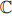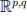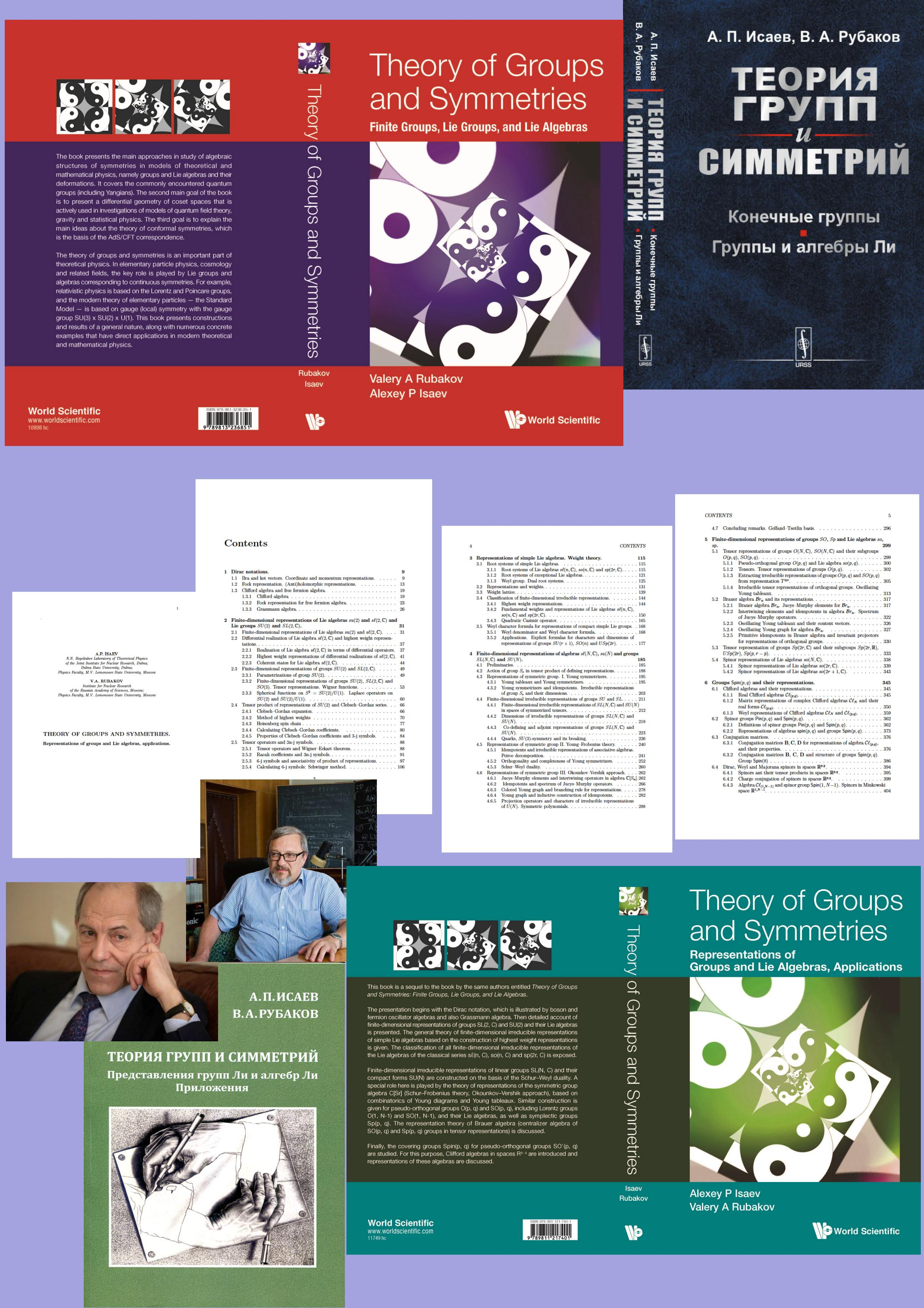# Theory of groups and symmetries. Representations of groups and Lie algebras. Applications

Publications, 08 September 2020

The First JINR Prize for 2019 in the section «Theoretical research» was awarded to Alexey Isaev (Bogoliubov Laboratory of Theoretical Physics of JINR, Dubna) and Valery Rubakov (RAS Institute for Nuclear Research, Moscow) for the series of studies “Theory of groups and symmetries. Representations of groups and Lie algebras. Applications”.

The first book  gives an extended exposition of constructions and results of the theory of groups and symmetries which have wide applications in theoretical and mathematical physics. The authors discuss both algebraic group theory and representation theory of Lie groups and algebras. Special emphasis is on compact Lie groups and algebras, as well on conformal groups and algebras in diverse dimensions. The classification of compact semi-simple finite-dimensional Lie algebras is given. Basis of Yangians related to simple Lie algebras of classical series is presented. The differential geometry of homogeneous spaces is studied.

This book is suitable for advanced undergraduate and graduate students as well as researchers in particle physics and mathematical physics.

The second book  is a sequel of the book  by the same authors. The presentation begins with the Dirac notation, which is illustrated by boson and fermion oscillator algebras and also Grassmann algebra. Then a detailed account of finite-dimensional representations of groups SL(2,) and SU(2) and their Lie algebras is presented. The general theory of finite-dimensional irreducible representations of simple Lie algebras based on the construction of the highest weight representations is given. The classification of all finite-dimensional irreducible representations of the Lie algebras of the classical series sℓ(n,), so(n,) and sp(2r,) is exposed. Finite dimensional irreducible representations of linear groups SL(N,) and their compact forms SU(N) are constructed on the basis of the Schur-Weyl duality. A special role here is played by the theory of representations of the symmetric group algebra[Sr] (Schur-Frobenius theory, Okounkov-Vershik approach), based on combinatorics of Young diagrams and Young tableaux. Similar construction is given for pseudo-orthoginal groups O(p, q) and SO(p, q), including Lorentz groups O(1, N − 1) and SO(1, N − 1), and their Lie algebras, as well as symplectic groups Sp(p, q). The representation theory of Brauer algebra (centralizer algebra of SO(p, q) and Sp(p, q) groups) is discussed.

Finally, the covering groups Spin(p, q) for pseudo-orthogonal groups SO (p, q) are studied. For this purpose, Clifford algebras in spacesare introduced and representations of these algebras are discussed.

 A.P.Isaev and V.A.Rubakov, Theory of Groups and Symmetries. Finite Groups, Lie Groups, and Lie Algebras, World Scientific Publishing (2018) 476 pages.

 A.P.Isaev and V.A.Rubakov, Theory of Groups and Symmetries. Representations of groups and Lie algebras, applications, World Scientific Publishing (2020) 600 pages.

Doctor of Physics and Mathematic A. P. Isaev, Deputy Director of BLTP JINR
RAS Academician V. A. Rubakov, Chief Scientific Researcher of INR RAS